Courses

# Physics Notes | EduRev

## Physics : Physics Notes | EduRev

The document Physics Notes | EduRev is a part of the Physics Course Basic Physics for IIT JAM.
All you need of Physics at this link: Physics

The Maxwell Relations

Modeling the dependence of the Gibbs and Helmholtz functions behave with varying temperature, pressure, and volume is fundamentally useful. But in order to do that, a little bit more development is necessary. To see the power and utility of these functions, it is useful to combine the First and Second Laws into a single mathematical statement. In order to do that, one notes that since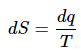(1)
for a reversible change, it follows that
dq = Tds                                                 (2)
And since
dw = Tds - pdV                                     (3)
for a reversible expansion in which only p-V works is done, it also follows that (since dU = dq + dw):

dU = Tds - pdV                                     (4)
This is an extraordinarily powerful result. This differential for dU can be used to simplify the differentials for H, A, and G. But even more useful are the constraints it places on the variables T, S, p, and V due to the mathematics of exact differentials!
Maxwell Relations
The above result suggests that the natural variables of internal energy are S and V (or the function can be considered as U(S,V)). So the total differential (dU) can be expressed: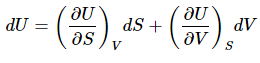(5)
Also, by inspection (comparing the two expressions for dU) it is apparent that: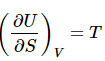(6)
and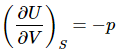(7)
But the value doesn’t stop there! Since dU is an exact differential, the Euler relation must hold that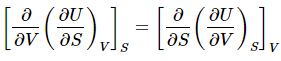(8)
or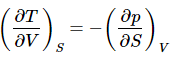(9)
This is an example of a Maxwell Relation. These are very powerful relationship that allows one to substitute partial derivatives when one is more convenient (perhaps it can be expressed entirely in terms of α and/or kT for example.)
A similar result can be derived based on the definition of H.
H = U + pV                                                                 (10)
Differentiating (and using the chain rule on (pV)) yields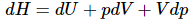(11)
Making the substitution using the combined first and second laws (dU=TdS–pdV) for a reversible change involving on expansion (p-V) work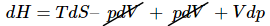(12)
This expression can be simplified by canceling the pdV terms.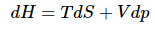(13)

And much as in the case of internal energy, this suggests that the natural variables of H are S and p. Or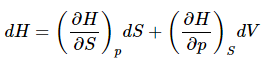(14)
Comparing equation 13 and 14 show that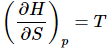(15)
and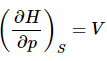(16)
It is worth noting at this point that both (equation 6)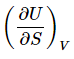(17)
and (Equation 15).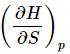(18)
are equation to T. So they are equation to each other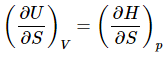(19)
Morevoer, the Euler Relation must also hold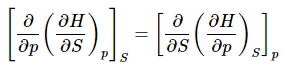(20)
This is the Maxwell relation on H. Maxwell relations can also be developed based on A and G. The results of those derivations are summarized in Table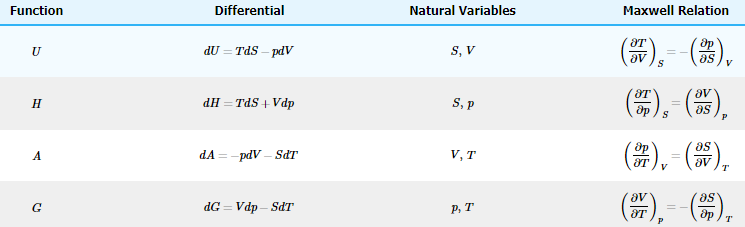The Maxwell relations are extraordinarily useful in deriving the dependence of thermodynamic variables on the state variables of p, T, and V.
Show that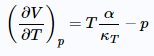(21)

dU = TdS - pdV                                              (22)
Divide both sides by dV and constraint to constant T: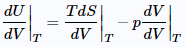(23)
Noting that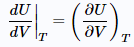(24)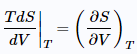(25)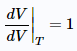(26)
The result is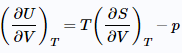Now, employ the Maxwell relation on Table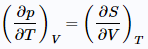to get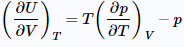and sinceIt is apparent that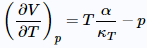Offer running on EduRev: Apply code STAYHOME200 to get INR 200 off on our premium plan EduRev Infinity!

,

,

,

,

,

,

,

,

,

,

,

,

,

,

,

,

,

,

,

,

,

;